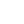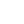Preview the Course
1h 8m

# Average Values for Constant Acceleration

by Robert Abramovic | Science FREE4 Lessons 1:08:29
1.1. Review of Constant Acceleration 8:15
2.2. Average Acceleration 3:18
3.3. Average Velocity 23:51
4.4. Average Position 33:05

1h 8m

NGSS Standards

FREE

Course

Constant acceleration is all around you. Every time you see an object fall, its acceleration due to gravity is constant. In this course, you will learn formulas for the average velocity and average position of an object accelerating at a constant rate. Both average velocity and average acceleration are computed in two ways: first by using a quotient and then by using an integral. In the final section, we define the average position using an integral. We then compute this integral and determine a formula for the average position as a function of time in terms of the initial position, final position, time elapsed, and acceleration.

Tags:

• Acceleration
• Kinematics
• Math
• Physics
• Science
• STEM
• Velocity

## Instructor

Hi, I'm Robert. I hold a Ph.D. in Mathematics as well as a BA in Physics. I have been a private tutor for over 13 years. I enjoying sharing my knowledge of mathematics and physics with students, creating relatable examples, and making learning fun. My philosophy is not only teaching students but inspiring them to learn more about the material on their own.

See full profile